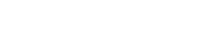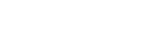# Spatial Data in .NET

Igor Marković, Software development consultant
25.11.2020.Conventional data in information systems mostly consists of text, numbers, dates and maybe some binary data. Spatial data is slightly less common. Spatial data contains information about geometrical properties of a certain entity, such as the position, length and surface area. This kind of data could theoretically also be stored using conventional data types, but it would be quite inconvenient for processing. Therefore, many database systems and software frameworks support using special datatypes for spatial data. In this post handling spatial data in the .NET Framework will be covered.

## Spatial Data Basics

Let’s start with a short and rather simplified overview of the basics of spatial data.

Spatial data is most often represented by the following distinct geometric objects:

• Point
• Line
• Polygon

A point is a single point in an area defined by its coordinates. A line is a list of points. A polygon is also a list of points, but it must be enclosed – the first and the last point have to match.

There is an ISO standard that defines textual representation of spatial data. It’s called Well Known Text – WKT. There is also a binary equivalent of WKT used for computer processing – Well Known Binary (WKB).

Here is an overview with examples that can be found on Wikipedia:

Generally, there are 2 types of coordinate systems:

• The plain 2D system
• The geodetic system, which takes the curviness of the Earth’s surface into consideration

## Using Spatial Data in .NET

In this example, a C# console application will be used.

In order to use spatial data, a reference System.Data.Entity assembly has to be added:

The corresponding using directive:

`using System.Data.Spatial;`

In .NET, the two spatial datatypes are:

• DbGeography //     Represents data in a geodetic (round earth) coordinate system.
• DbGeometry //     Provides a base class for objects that define geometric shapes.

We will use DbGeography in this example. By default, coordinates in DbGeography represent geographical longitude and latitude.

We will use the following three entities:

• Shops – defined by location, i.e. Point
• Roads – defined by start, intermediate and end points, i.e. Line
• Parks – defined by boundaries, i.e. Polygon

Additionally, we will be defining a random point as “my location”.

Here’s an approximate visualisation of the example that will be used:

So, let’s get to coding.

The base class for spatial entities:

`public abstract class SpatialEntity`
`{`
`    public string Name { get; set; }`
`    public DbGeography Location { get; set; }`
`}`
`The corresponding derived classes with an appropriate additional property:`
`public class Shop : SpatialEntity`
`{`
`        public string OpenHours { get; set; }`
`}`
`public class Road : SpatialEntity`
`{`
`       public string SurfaceType { get; set; }`
`}`
`public class Park : SpatialEntity`
`{`
`    public bool HasLakes { get; set; }`
`}`

The area will be placed around 50 degrees longitude and 0 degrees latitude – that's where London is approximately located.

The WKT syntax for points:

`POINT (<longtitude> <latitude>)`

Let's create our area according to the previously shown image:

`List spatialEntities = new List();`
`            //shops`
`            spatialEntities.Add(`
`                new Shop { Name = "Shop 1",`
`                Location = DbGeography.FromText("POINT (0.00110 50.00200)"),`
`                OpenHours = "08-20" });`
`            spatialEntities.Add(`
`                new Shop { Name = "Shop 2",`
`                Location = DbGeography.FromText("POINT (0.00190 50.00100)"),`
`                OpenHours = "08-18" });`
`            spatialEntities.Add(`
`                new Shop { Name = "Shop 3",`
`                Location = DbGeography.FromText("POINT (0.00090 50.00140)"),`
`                OpenHours = "08-16" });`
`            //roads`
`            spatialEntities.Add(`
`                new Road { Name = "Road 1",`
`                Location = DbGeography.FromText("LINESTRING (0.00110 50.00200, 0.00210 50.00110, 0.00220 50.00190, 0.00320 50.00080)"),`
`                SurfaceType = "Tarmac" });`
`            spatialEntities.Add(`
`                new Road { Name = "Road 2",`
`                Location = DbGeography.FromText("LINESTRING (0.00120 50.0010, 0.00130 50.0020, 0.00140 50.0010, 0.00150 50.00040)"),`
`                SurfaceType = "Gravel" });`
`            //park`
`            spatialEntities.Add(`
`                new Park { Name = "The park",`
`                Location = DbGeography.FromText("POLYGON ((0.00200 50.00300, 0.00200 50.00010, 0.00250 50.00010, 0.00200 50.00300))"),`
`                HasLakes = false });`
`//my location`
`            DbGeography myLocation = DbGeography.FromText("POINT (0.00100 50.00150)");`

A typical spatial query is finding the closest point to a certain location. So, let’s find the answer to the question what the closest shop is. The answer will be provided by a LINQ query and Distance() method:

`//What is the closest shop?`
`            var chosestShop = spatialEntities`
`                                    .Where(x => x is Shop)`
`                                    .OrderBy(x => x.Location.Distance(myLocation))`
`                                    .FirstOrDefault();`
`            Console.WriteLine(\$"The closest shop to you is: {chosestShop.Name}"); //Shop 3`
`Let’s have another example. Let’s find out which road passes through the park. The answer will be provided by a LINQ query and Intersects() method:`
`//Which road passes through the park?`
`            Park thePark = spatialEntities.First(x => x is Park) as Park;`
`            var roadThroughPark = spatialEntities`
`                                    .Where(x => x is Road && x.Location.Intersects(thePark.Location))`
`                                    .FirstOrDefault();`
`            Console.WriteLine(\$"The road which passes through the park: {roadThroughPark.Name}");  //Road 1`

Persistence

In order to handle persistent data from a database, using Entity Framework 5 or newer is required.

Wrapping Up

The .NET Framework provides quite a convenient way of handling spatial data, allowing the usage of standard spatial operations such as “Distance”, “Intersects”, etc. Using EF5 or newer, you can also handle persistent data from a database.